# Inverse of Euclidean distance?

3399
13
02-12-2018 05:06 PMby
New Contributor II
Hi everyone,
I am trying to do the reverse / inverse of a euclidean distance. For example, I am doing distance to roads analysis. The output from the distance raster shows roads as 0 and areas closest to roads as 0.1, 0.2, etc and the areas furthest from roads as a very high value. However, I would like the raster to be the reverse of this - the areas closest to the roads should have the highest values and the areas furthest from the roads should have the lowest values.  Specifically, the areas closest to the roads are 0.001 and the areas furthest from the roads are 3000. I would like the reverse - the areas closest to the roads to be 3000 and the areas furthest from the roads to be 0.001. How do I create a raster that reverses the number?
I would use reclass but this would be difficult to use as the values are continuous and not integers. Is there a way to do this in reclass or raster calculator that I am not aware of for continuous values without overgeneralizing them? Or is there a different tool that I can use?
I am trying to do this in IDRISI so if any of you know how to do it in this program, that would be exceedingly helpful. If not, I am definitely happy with ideas on how to do this in ArcGIS, and then I can import the file into IDRISI after editing it in ArcGIS.
Thank you!
Tags (4)
1 Solution

Accepted SolutionsbyEsri Esteemed Contributor

And I am sorry that I wasn't any clearer with my explanation, since it does exactly what I believe you are requesting. Just to be sure, open the properties of the distance raster and post back the minimum and maximum values. Although you mention that they are 0.001 and  3000, I'm sure they will be 0 to something around 3000. Knowing the exact values is important to avoid errors. And perhaps good to ask, are you using ArcMap or ArcGIS Pro (and please provide the version of the software you are using)?

Let's just assume that you really do have values in the range from 0.001 to 3000 and you want them to be 3000 to 0.001. Then you could follow these steps to determine the formula:

Input rangeApplyOutput range
0.001 to 3000value - 0.0010 to 2999.999
0 to 2999.999value / 2999.9990 to 1
0 to 11 - value1 to 0
1 to 0* 2999.9992999.999 to 0
2999.999 to 0+ 0.0013000 to 0.001

``(1 - ((Raster - 0.001) / 2999.999)) * 2999.999 + 0.001‍‍‍‍``

Or more general:

``(1 - ((Raster - min) / (max - min))) * (max - min) + min‍‍‍‍``
13 RepliesbyMVP Esteemed Contributor

if you want the inverse of the Euclidean distance, the first thing you have to do is assign all your 0's to nodata, then you can create a constant raster with the exact same cell size extent etc etc as the Euclidean raster, then do a big divide..

Of course you can do it other ways, but this way, you get to use a few tools that you probably haven't used before

If you then need an integer grid or need to reclass... do it afterby
New Contributor II

Thanks Dan! What value would you suggest that I give the constant raster? Sorry but I am a little bit unclear as to how this might help solve the problem. If I divide the Euclidean distance by a constant raster (ie let's say I divide everything by 2), then I would still have the areas that are furthest away be the highest values and not the lowest values - they would all just be half the value that they were before. Maybe I am understanding this completely wrong? Can you please let me know what I am not understanding?byMVP Esteemed Contributor

one... 1... 1/something... ie  1/10 is 0.1   then you can scale up your result to whatever number you want.

Some are suggesting the maximum value, but there is no need, unless you need to use the inverse Euclidean distance as a scaling or multiplicative factor in some overall cost scheme.

Just experiment... Xander has some pictoral examples, but you are the one that has to use the actual data.  So decide what values you really need.byEsri Contributor

Hi Amy,

Interesting question. The answer depends on which highest value you want to assign closest to the roads? If you want to assign the maximum value from the Euclidean Distance output next to all of your roads, then you just have to do a simple minus.

Conceptually:

reverseEucDistance = maximum_distance_as_a_number - outputFromEuclideanDisntace.

The problem with this is, the farthest value from each road will not be zero. So you can try the following alternative.

If your roads have unique values, instead of running Euclidean Distance tool, please run Euclidean Allocation tool and create output allocation raster and output distance raster.

Now run ZonalStatistics tool with output allocation raster as zone, output distance raster as value raster, and select Maximum as the statistics type.

Now do a simple minus again as follows:

reverseEucDistance = ZonalStatistics_output - output_distance_raster_from_EuclideanAlloction.

Hopefully, these steps will give you the result you are expecting.

Thanks,by
New Contributor II

Thank you Nawajish! My roads do not have unique values - they are all the same in terms of how influential they are to the analysis. At the moment, roads are labeled 1 and the background of the raster is 0. I tried running Euclidean Allocation on this and it didn't work. I tried using the FID unique value as the source field and running Euclidean Allocation again and this did not work either. Can you please let me know what you mean by what kind of unique values the roads should have in order to run the Euclidean Allocation tool? Thanks!byEsri Esteemed Contributor

I you just want to invert the distance, you would have to calculate the highest value minus the distance raster. So do your euclidean distance: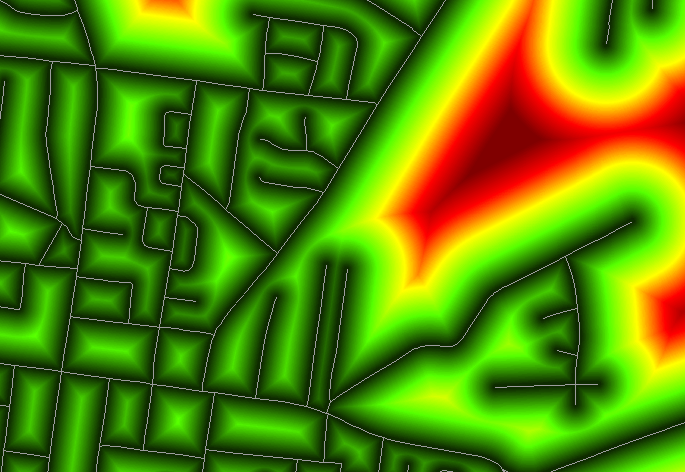Go into the properties of the raster and read out the maximum value: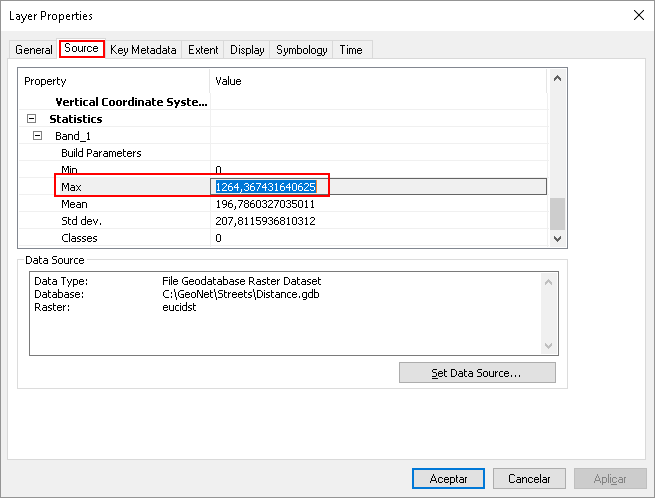Go into the Raster Calculator:... and execute the calculation: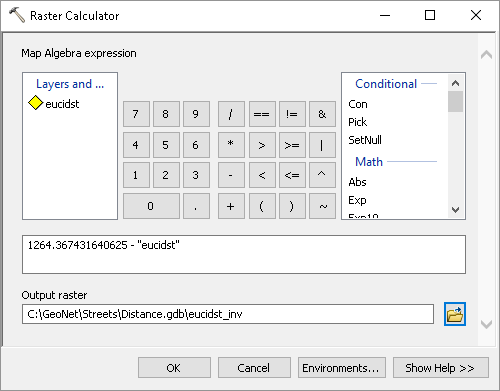(replacing the maximum value by your maximum value) and you will have inverted your raster: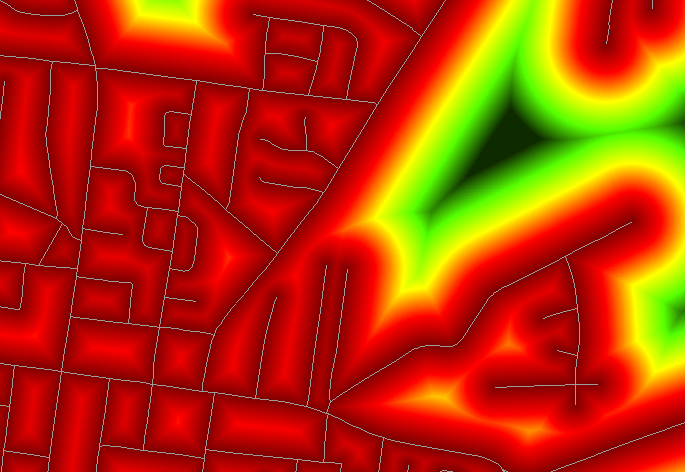Common use case to do this is to set for instance a score based on distance of the roads, where close to the streets might represent a positive effect (higher score) and further away should,receive a lower score.

You could do this in a single step on your distance raster. See below how the calculation to be executed is determined. In this case my input distance value range is approximately 0 - 1265 and I would like to assign a range of 10 - 1 to these values:

Input rangeApplyOutput range
0 - 1265value / 12650 - 1
0 - 11 - value1 - 0
1 - 0value * 99 - 0
9 - 0value + 110 - 1

The actual formula would become:

``(1 - (Raster / 1265)) * 9  + 1‍``

If I execute the formula:I get this range (the 1.0045 is due to not using the exact value):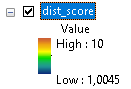You can enhance the calculation including ranges of value that should be set to the minimum or maximum score by using Con statements.by
New Contributor II

Hi Xander! Thank you so much for all of your suggestions! They would be insanely helpful except for unfortunately this is not what I would like to do. I guess I was a bit unclear when I said that I wanted to create an "inverse." Essentially, the current raster contains areas closest to roads as smaller values and the areas furthest from roads as a very high value. However, I would like the raster to be the reverse of this - the areas closest to the roads should have the highest values and the areas furthest from the roads should have the lowest values. Specifically, the areas closest to the roads are 0.001 and the areas furthest from the roads are 3000. I would like the reverse - the areas closest to the roads to be 3000 and the areas furthest from the roads to be 0.001. Unfortunately for the analysis that I am doing, I can't have any negative numbers and they must be continuous, not integers.

Thanks so much for taking the time to create all of the images and step-by-step instructions. That was so kind of you! I apologize that I wasn't clear enough in my question.byEsri Esteemed Contributor

And I am sorry that I wasn't any clearer with my explanation, since it does exactly what I believe you are requesting. Just to be sure, open the properties of the distance raster and post back the minimum and maximum values. Although you mention that they are 0.001 and  3000, I'm sure they will be 0 to something around 3000. Knowing the exact values is important to avoid errors. And perhaps good to ask, are you using ArcMap or ArcGIS Pro (and please provide the version of the software you are using)?

Let's just assume that you really do have values in the range from 0.001 to 3000 and you want them to be 3000 to 0.001. Then you could follow these steps to determine the formula:

Input rangeApplyOutput range
0.001 to 3000value - 0.0010 to 2999.999
0 to 2999.999value / 2999.9990 to 1
0 to 11 - value1 to 0
1 to 0* 2999.9992999.999 to 0
2999.999 to 0+ 0.0013000 to 0.001

``(1 - ((Raster - 0.001) / 2999.999)) * 2999.999 + 0.001‍‍‍‍``
``(1 - ((Raster - min) / (max - min))) * (max - min) + min‍‍‍‍``by